Скачать презентацию N Gregory Mankiw Power Point Slides by Ron

26fe034fa2d25dd784d73fb00b402df5.ppt

• Количество слайдов: 49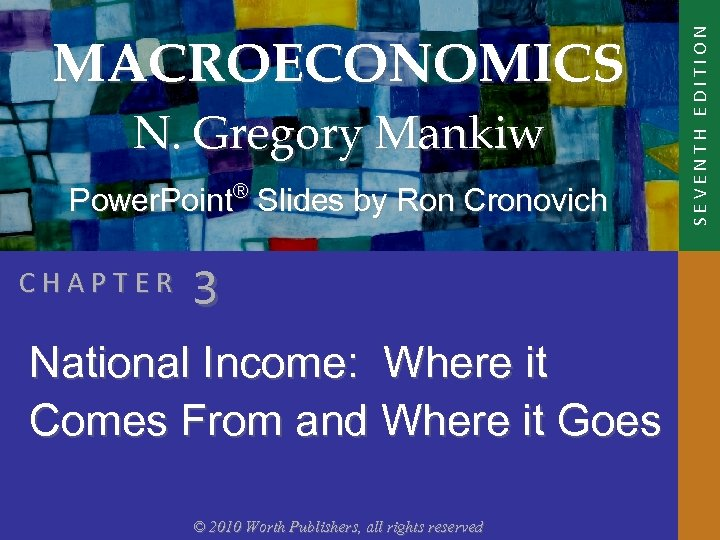N. Gregory Mankiw Power. Point® Slides by Ron Cronovich CHAPTER 3 National Income: Where it Comes From and Where it Goes © 2010 Worth Publishers, all rights reserved SEVENTH EDITION MACROECONOMICS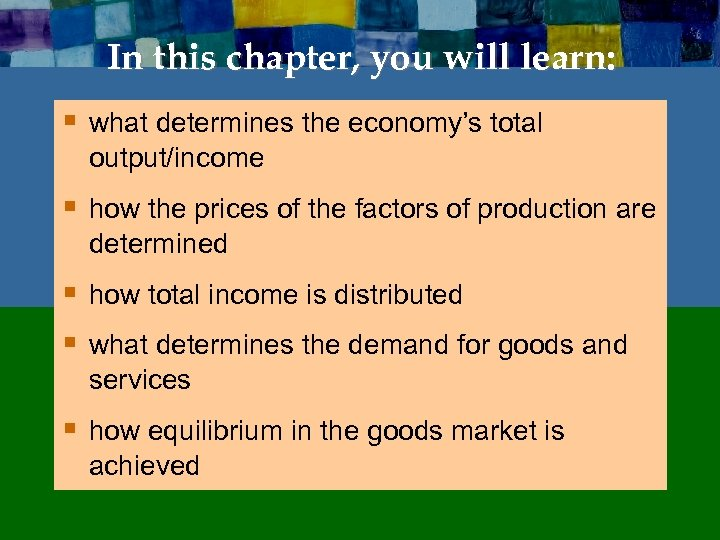In this chapter, you will learn: § what determines the economy’s total output/income § how the prices of the factors of production are determined § how total income is distributed § what determines the demand for goods and services § how equilibrium in the goods market is achieved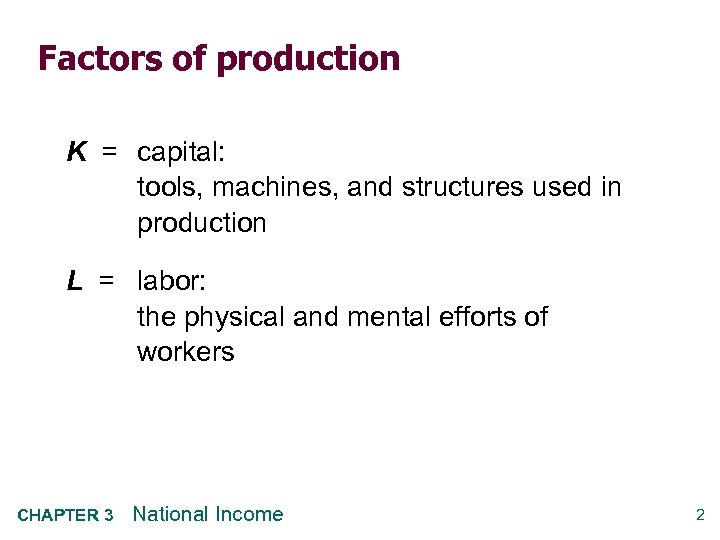Factors of production K = capital: tools, machines, and structures used in production L = labor: the physical and mental efforts of workers CHAPTER 3 National Income 2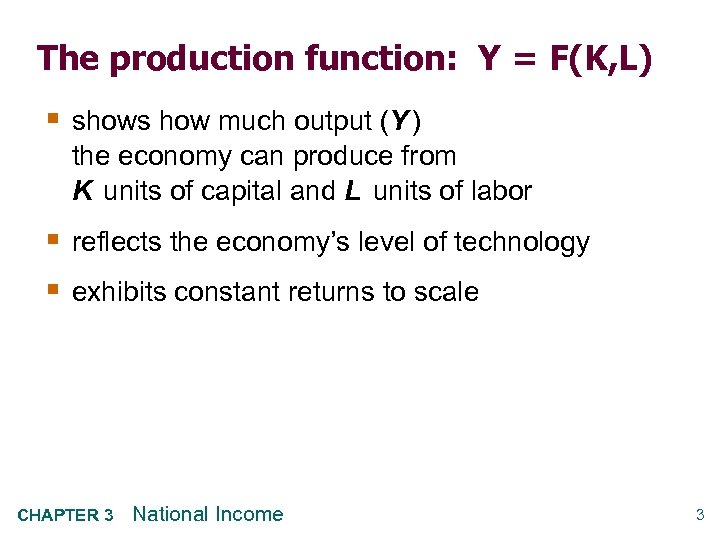The production function: Y = F(K, L) § shows how much output (Y ) the economy can produce from K units of capital and L units of labor § reflects the economy’s level of technology § exhibits constant returns to scale CHAPTER 3 National Income 3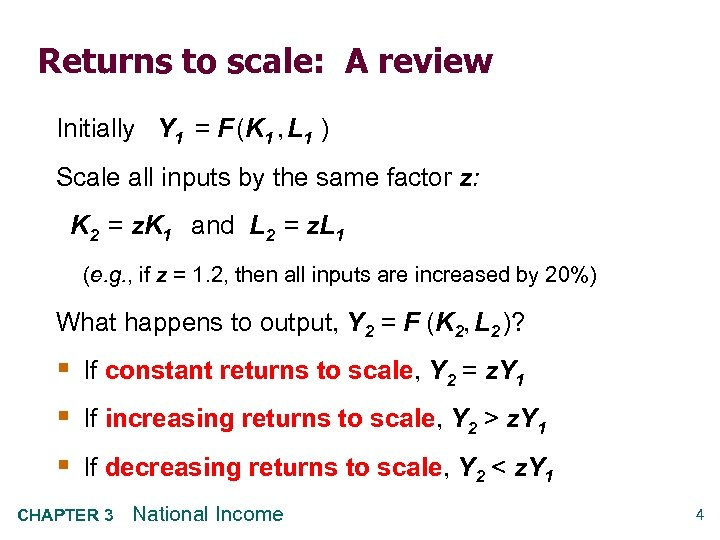Returns to scale: A review Initially Y 1 = F (K 1 , L 1 ) Scale all inputs by the same factor z: K 2 = z. K 1 and L 2 = z. L 1 (e. g. , if z = 1. 2, then all inputs are increased by 20%) What happens to output, Y 2 = F (K 2, L 2 )? § If constant returns to scale, Y 2 = z. Y 1 § If increasing returns to scale, Y 2 > z. Y 1 § If decreasing returns to scale, Y 2 < z. Y 1 CHAPTER 3 National Income 4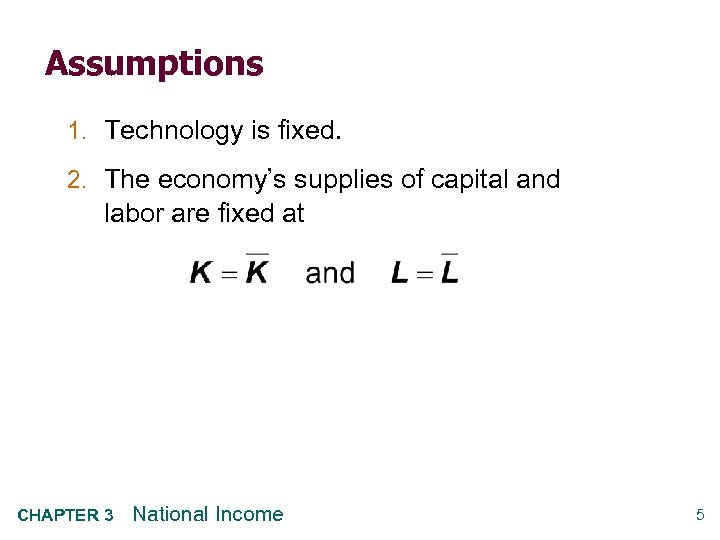Assumptions 1. Technology is fixed. 2. The economy’s supplies of capital and labor are fixed at CHAPTER 3 National Income 5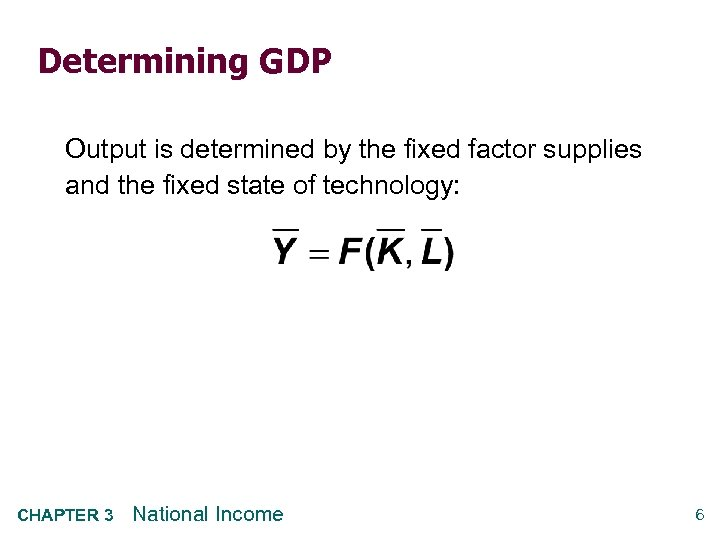Determining GDP Output is determined by the fixed factor supplies and the fixed state of technology: CHAPTER 3 National Income 6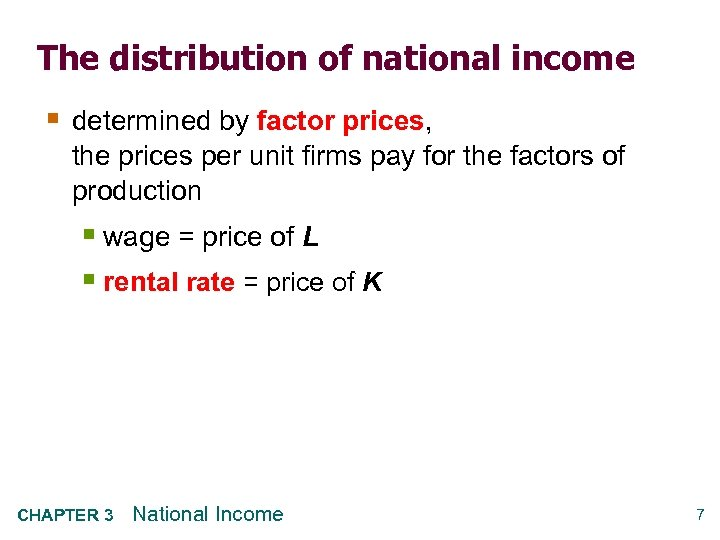The distribution of national income § determined by factor prices, the prices per unit firms pay for the factors of production § wage = price of L § rental rate = price of K CHAPTER 3 National Income 7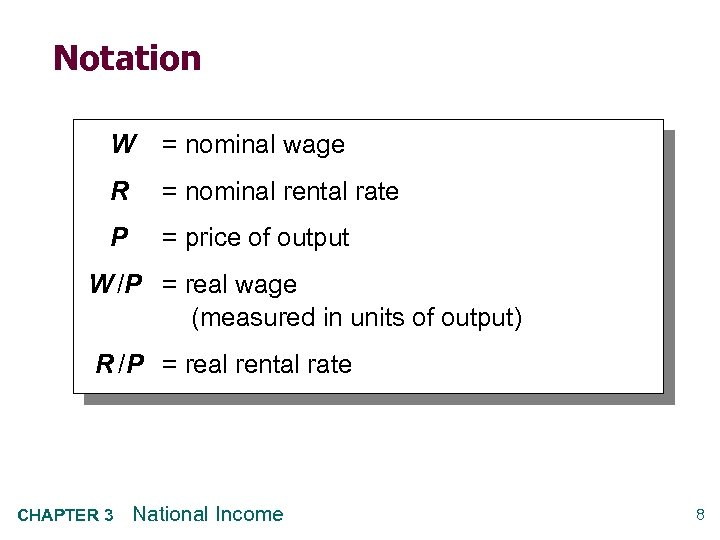Notation W = nominal wage R = nominal rental rate P = price of output W /P = real wage (measured in units of output) R /P = real rental rate CHAPTER 3 National Income 8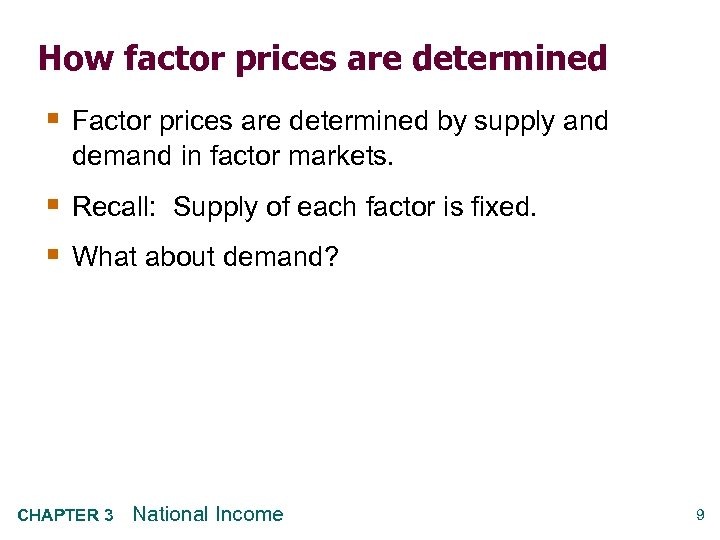How factor prices are determined § Factor prices are determined by supply and demand in factor markets. § Recall: Supply of each factor is fixed. § What about demand? CHAPTER 3 National Income 9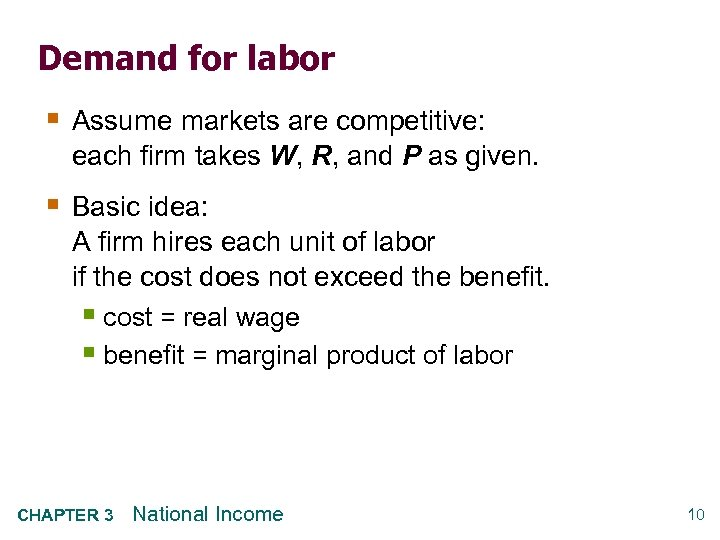Demand for labor § Assume markets are competitive: each firm takes W, R, and P as given. § Basic idea: A firm hires each unit of labor if the cost does not exceed the benefit. § cost = real wage § benefit = marginal product of labor CHAPTER 3 National Income 10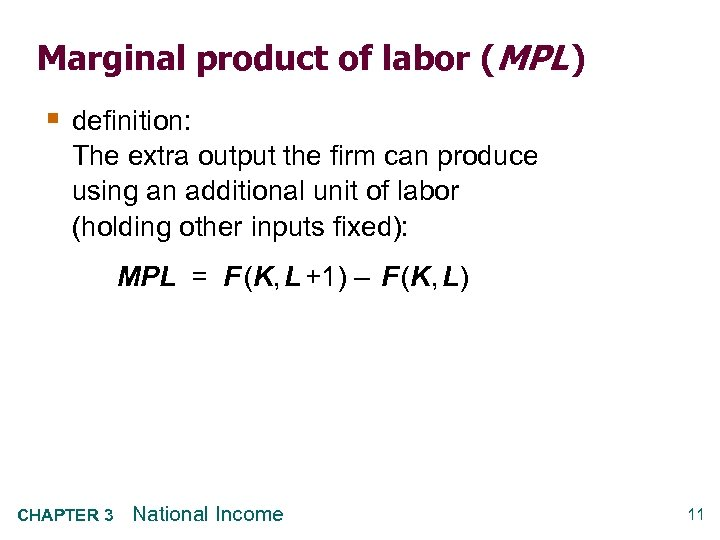Marginal product of labor (MPL ) § definition: The extra output the firm can produce using an additional unit of labor (holding other inputs fixed): MPL = F (K, L +1) – F (K, L) CHAPTER 3 National Income 11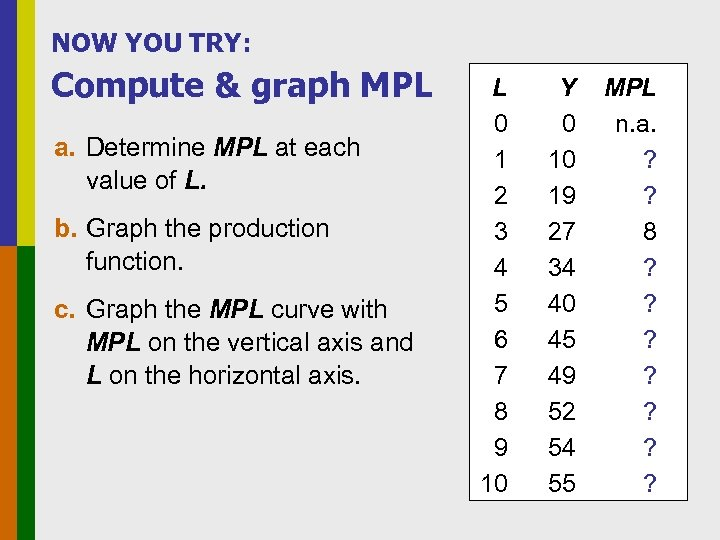NOW YOU TRY: Compute & graph MPL a. Determine MPL at each value of L. b. Graph the production function. c. Graph the MPL curve with MPL on the vertical axis and L on the horizontal axis. L 0 1 2 3 4 5 6 7 8 9 10 Y 0 10 19 27 34 40 45 49 52 54 55 MPL n. a. ? ? 8 ? ? ? ?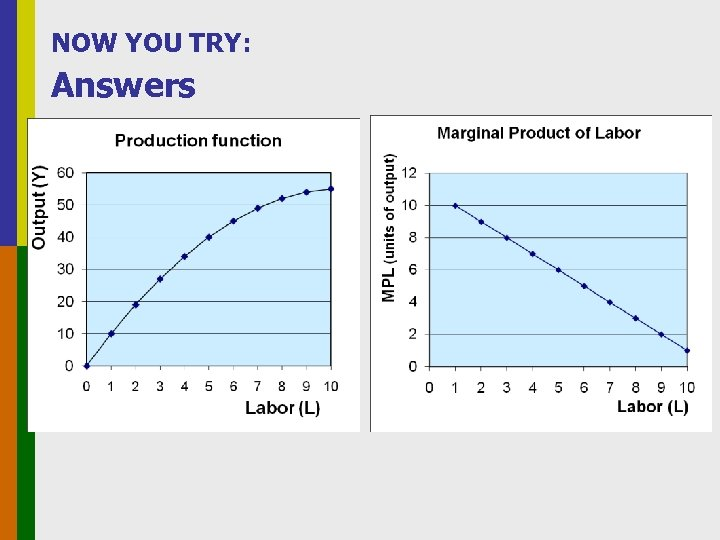NOW YOU TRY: Answers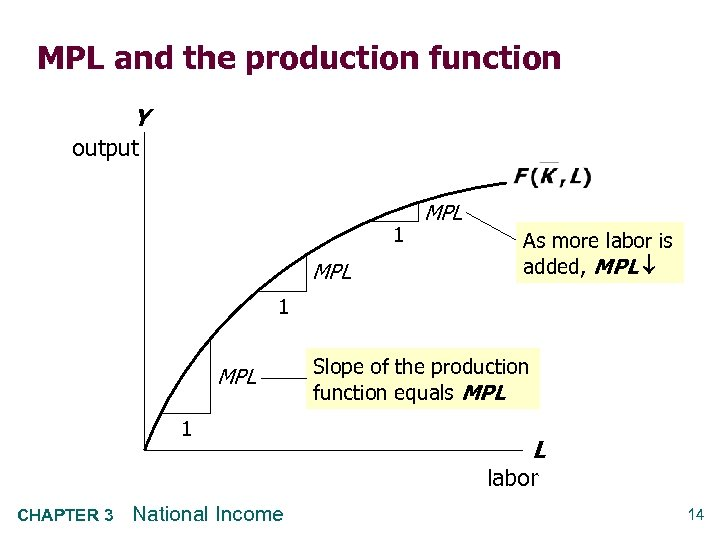MPL and the production function Y output 1 MPL As more labor is added, MPL 1 MPL 1 Slope of the production function equals MPL L labor CHAPTER 3 National Income 14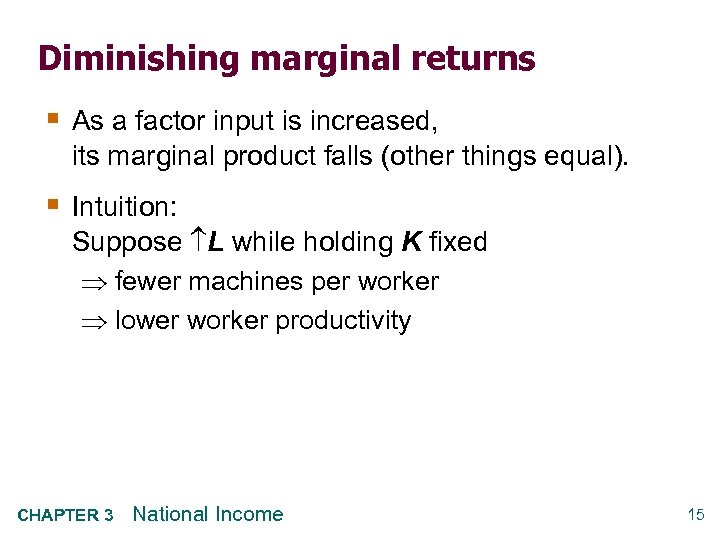Diminishing marginal returns § As a factor input is increased, its marginal product falls (other things equal). § Intuition: Suppose L while holding K fixed fewer machines per worker lower worker productivity CHAPTER 3 National Income 15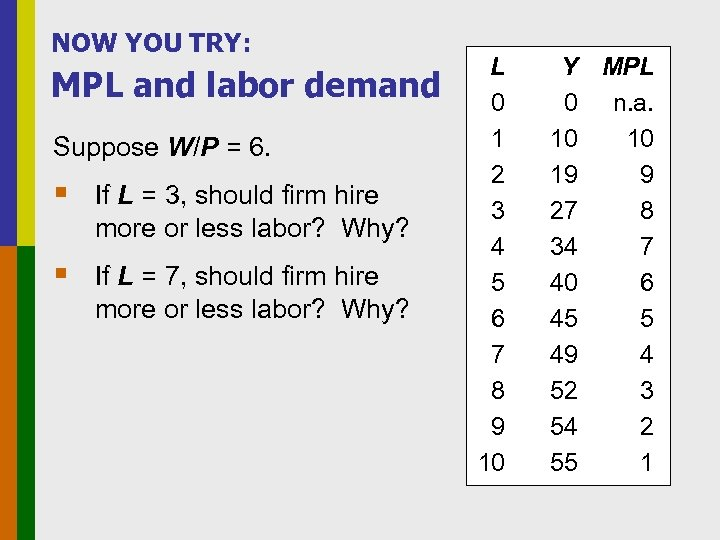NOW YOU TRY: MPL and labor demand Suppose W/P = 6. § If L = 3, should firm hire more or less labor? Why? § If L = 7, should firm hire more or less labor? Why? L 0 1 2 3 4 5 6 7 8 9 10 Y MPL 0 n. a. 10 10 19 9 27 8 34 7 40 6 45 5 49 4 52 3 54 2 55 1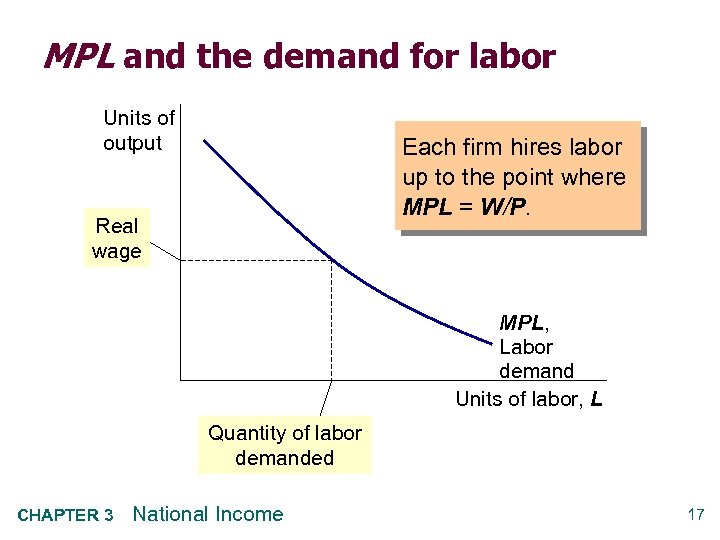MPL and the demand for labor Units of output Each firm hires labor up to the point where MPL = W/P. Real wage MPL, Labor demand Units of labor, L Quantity of labor demanded CHAPTER 3 National Income 17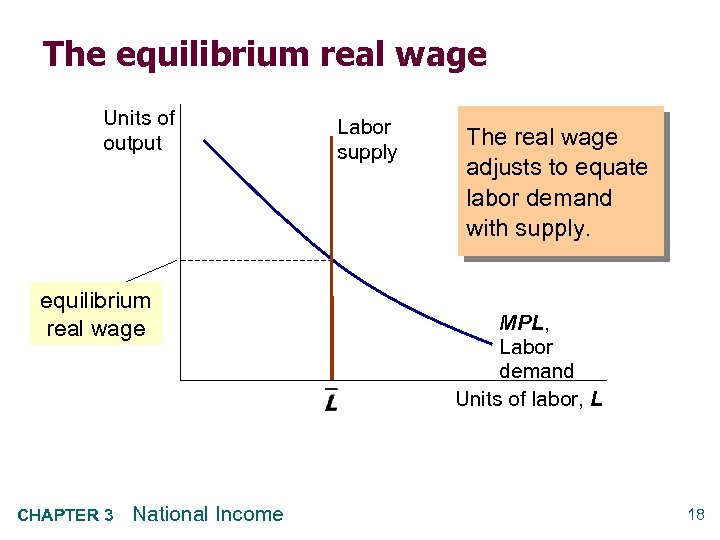The equilibrium real wage Units of output equilibrium real wage CHAPTER 3 National Income Labor supply The real wage adjusts to equate labor demand with supply. MPL, Labor demand Units of labor, L 18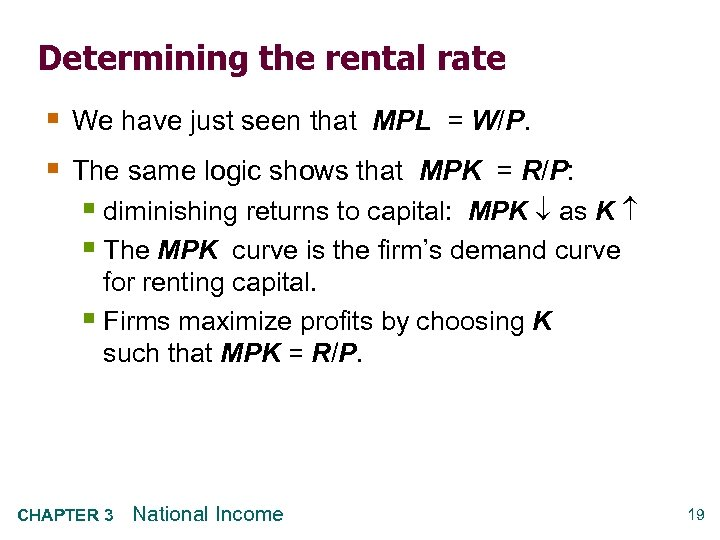Determining the rental rate § We have just seen that MPL = W/P. § The same logic shows that MPK = R/P: § diminishing returns to capital: MPK as K § The MPK curve is the firm’s demand curve for renting capital. § Firms maximize profits by choosing K such that MPK = R/P. CHAPTER 3 National Income 19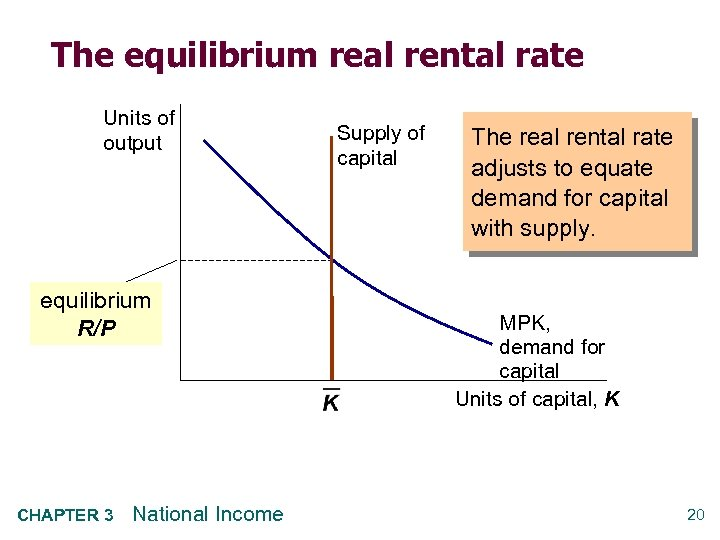The equilibrium real rental rate Units of output equilibrium R/P CHAPTER 3 National Income Supply of capital The real rental rate adjusts to equate demand for capital with supply. MPK, demand for capital Units of capital, K 20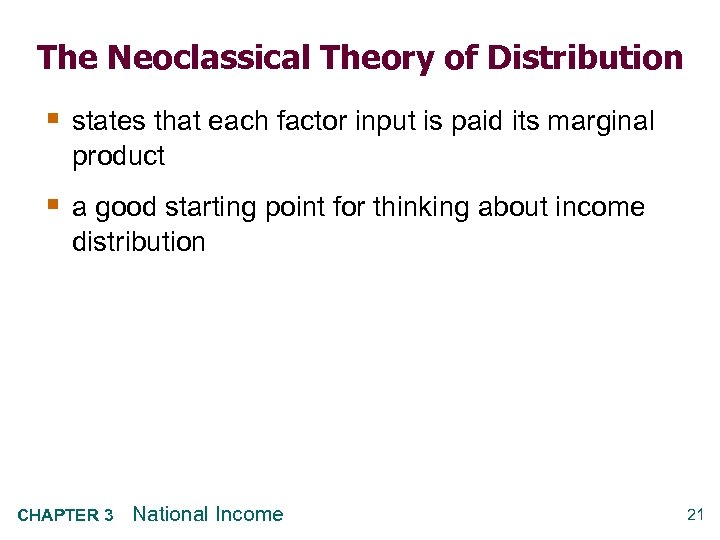The Neoclassical Theory of Distribution § states that each factor input is paid its marginal product § a good starting point for thinking about income distribution CHAPTER 3 National Income 21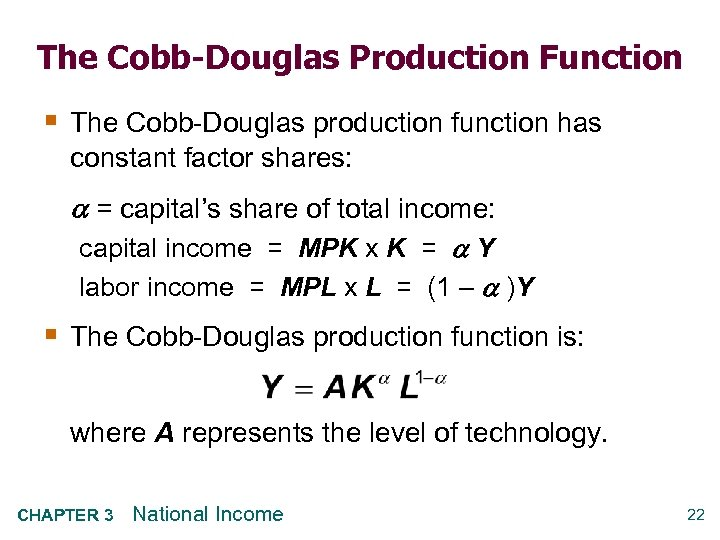The Cobb-Douglas Production Function § The Cobb-Douglas production function has constant factor shares: = capital’s share of total income: capital income = MPK x K = Y labor income = MPL x L = (1 – )Y § The Cobb-Douglas production function is: where A represents the level of technology. CHAPTER 3 National Income 22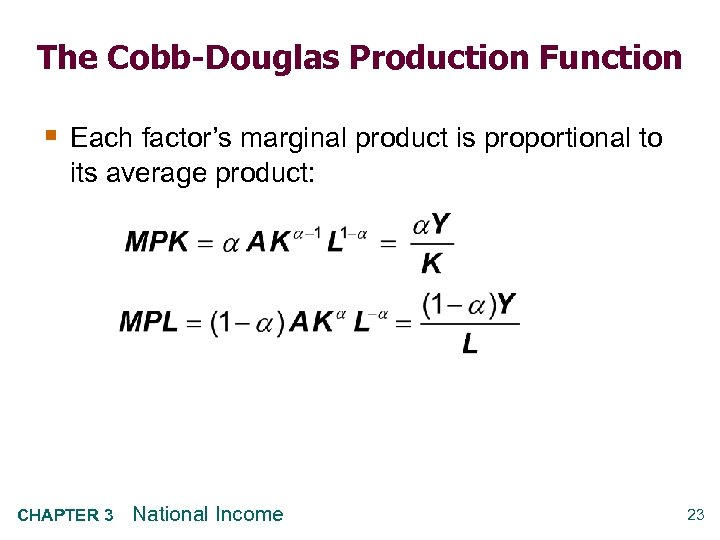The Cobb-Douglas Production Function § Each factor’s marginal product is proportional to its average product: CHAPTER 3 National Income 23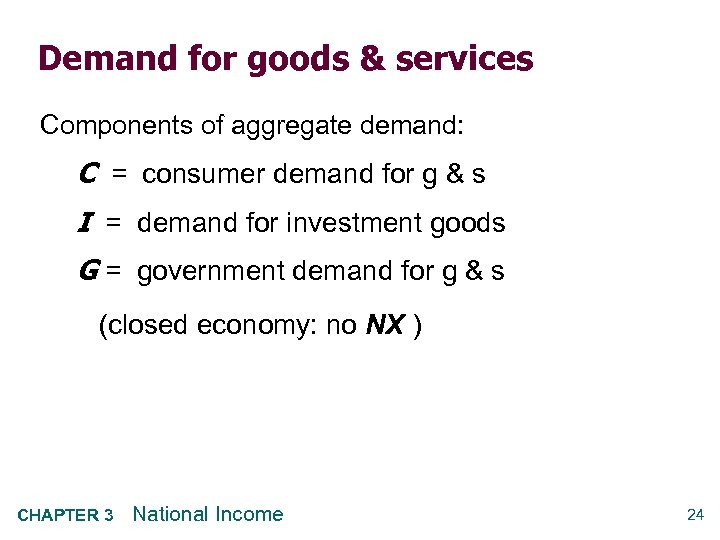Demand for goods & services Components of aggregate demand: C = consumer demand for g & s I = demand for investment goods G = government demand for g & s (closed economy: no NX ) CHAPTER 3 National Income 24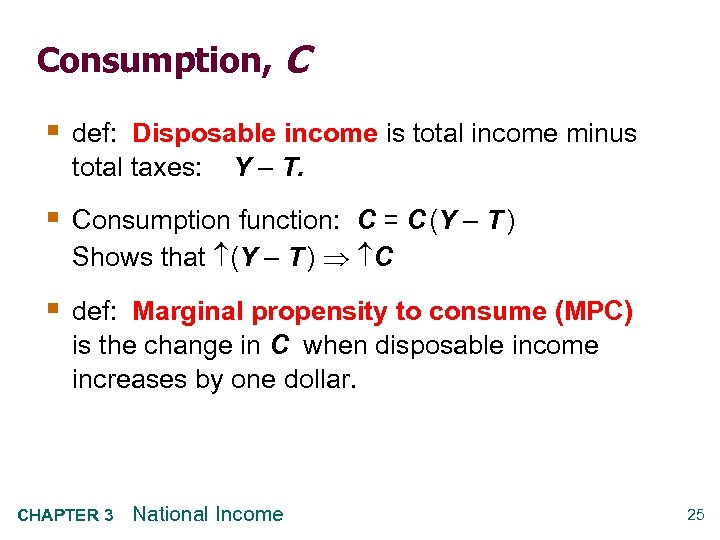Consumption, C § def: Disposable income is total income minus total taxes: Y – T. § Consumption function: C = C (Y – T ) Shows that (Y – T ) C § def: Marginal propensity to consume (MPC) is the change in C when disposable income increases by one dollar. CHAPTER 3 National Income 25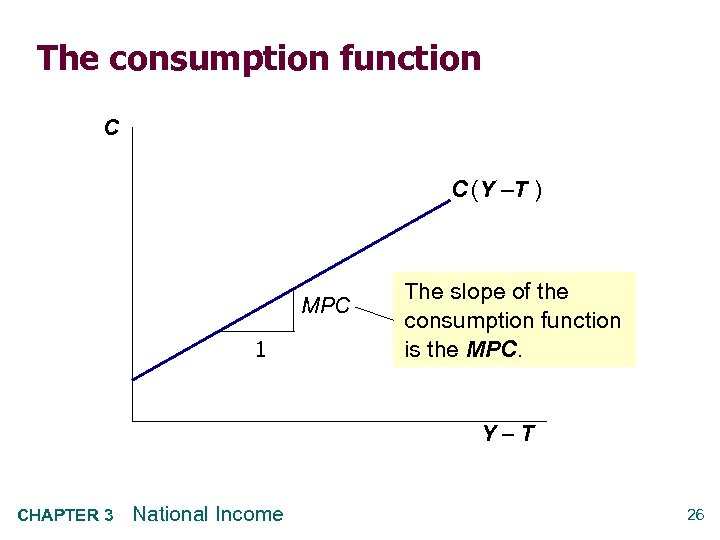The consumption function C C (Y –T ) MPC 1 The slope of the consumption function is the MPC. Y–T CHAPTER 3 National Income 26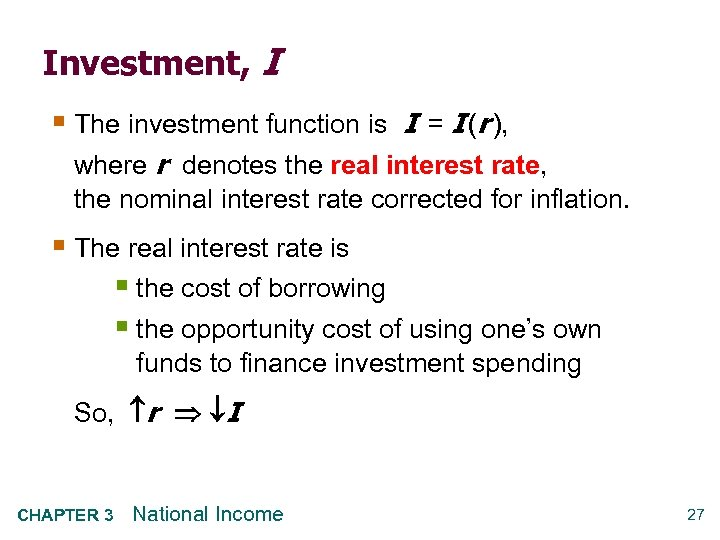Investment, I § The investment function is I = I (r ), where r denotes the real interest rate, the nominal interest rate corrected for inflation. § The real interest rate is § the cost of borrowing § the opportunity cost of using one’s own funds to finance investment spending So, r I CHAPTER 3 National Income 27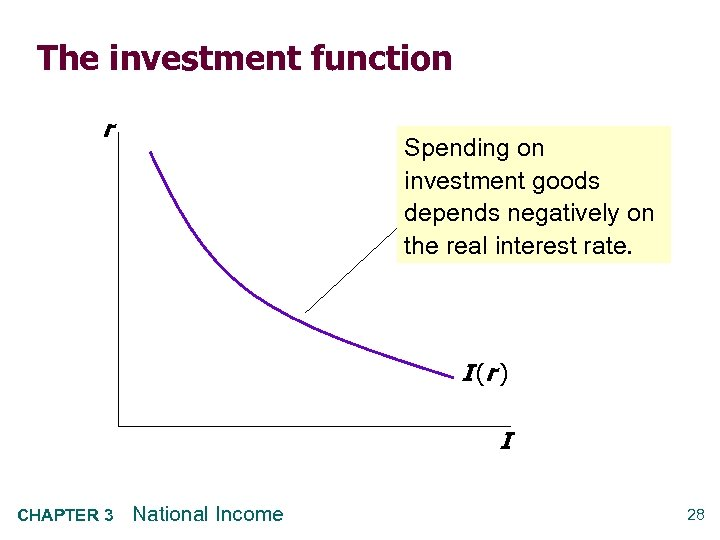The investment function r Spending on investment goods depends negatively on the real interest rate. I (r ) I CHAPTER 3 National Income 28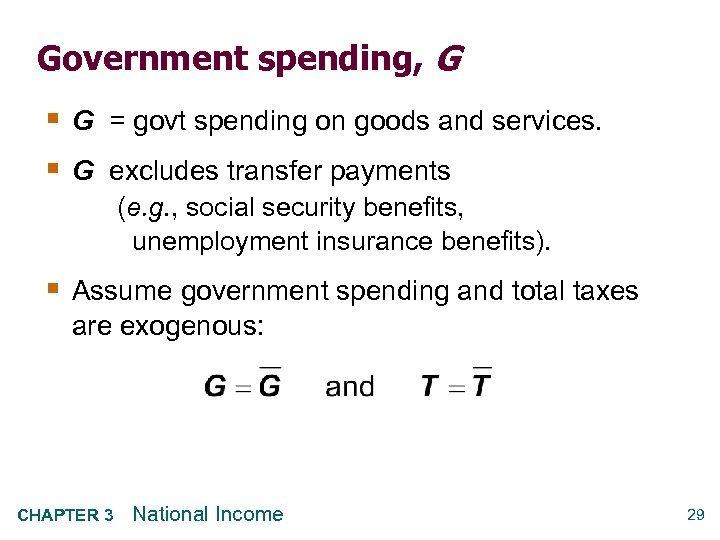Government spending, G § G = govt spending on goods and services. § G excludes transfer payments (e. g. , social security benefits, unemployment insurance benefits). § Assume government spending and total taxes are exogenous: CHAPTER 3 National Income 29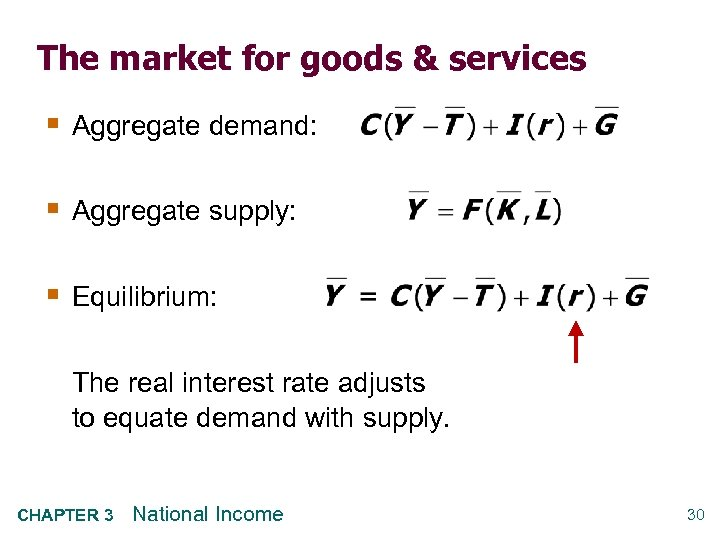The market for goods & services § Aggregate demand: § Aggregate supply: § Equilibrium: The real interest rate adjusts to equate demand with supply. CHAPTER 3 National Income 30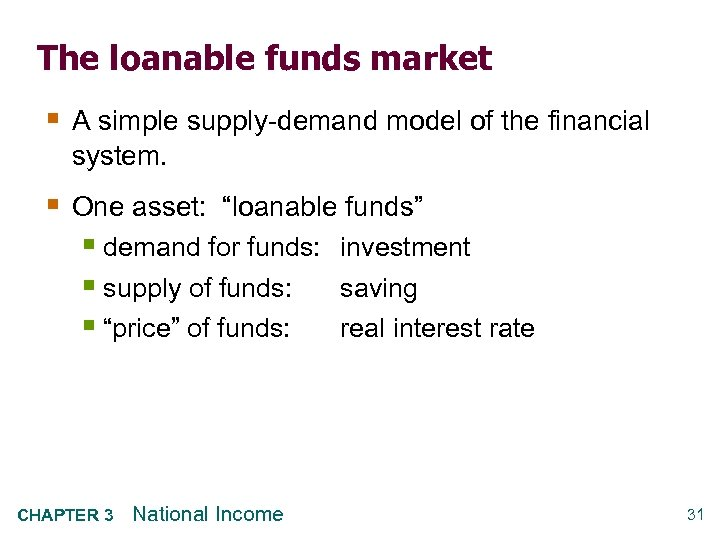The loanable funds market § A simple supply-demand model of the financial system. § One asset: “loanable funds” § demand for funds: investment § supply of funds: saving § “price” of funds: real interest rate CHAPTER 3 National Income 31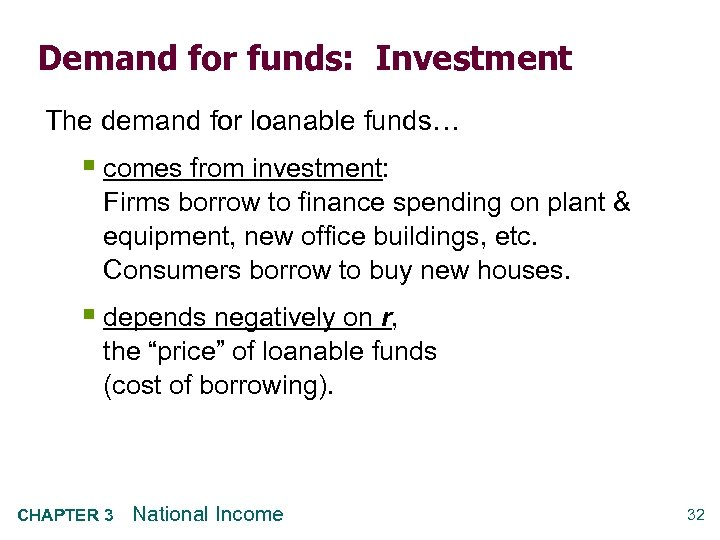Demand for funds: Investment The demand for loanable funds… § comes from investment: Firms borrow to finance spending on plant & equipment, new office buildings, etc. Consumers borrow to buy new houses. § depends negatively on r, the “price” of loanable funds (cost of borrowing). CHAPTER 3 National Income 32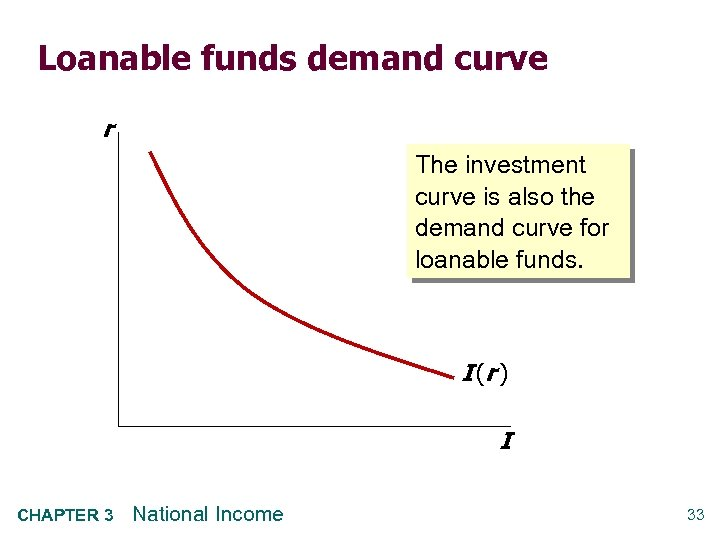Loanable funds demand curve r The investment curve is also the demand curve for loanable funds. I (r ) I CHAPTER 3 National Income 33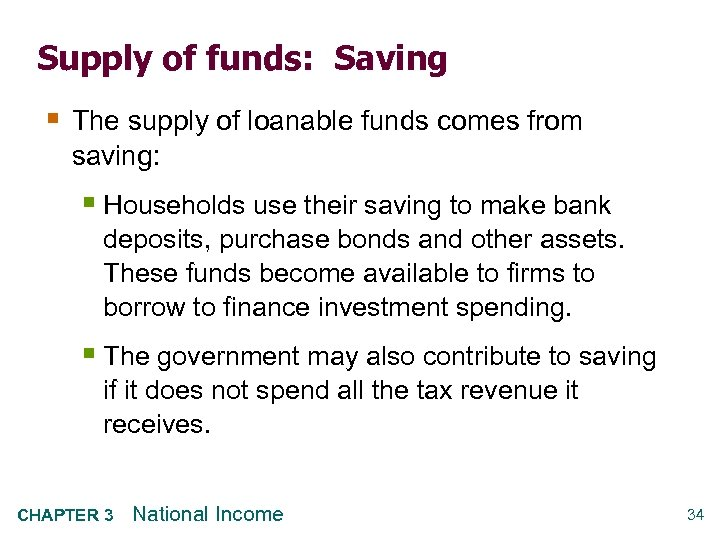Supply of funds: Saving § The supply of loanable funds comes from saving: § Households use their saving to make bank deposits, purchase bonds and other assets. These funds become available to firms to borrow to finance investment spending. § The government may also contribute to saving if it does not spend all the tax revenue it receives. CHAPTER 3 National Income 34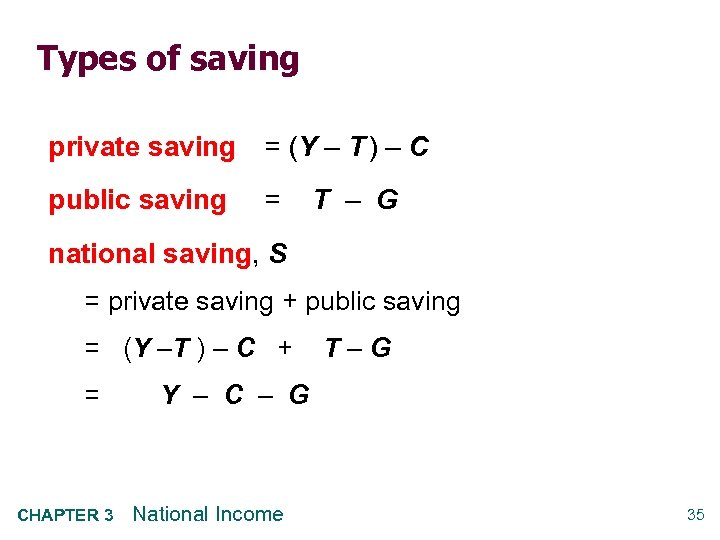Types of saving private saving = (Y – T ) – C public saving = T – G national saving, S = private saving + public saving = (Y –T ) – C + = CHAPTER 3 T–G Y – C – G National Income 35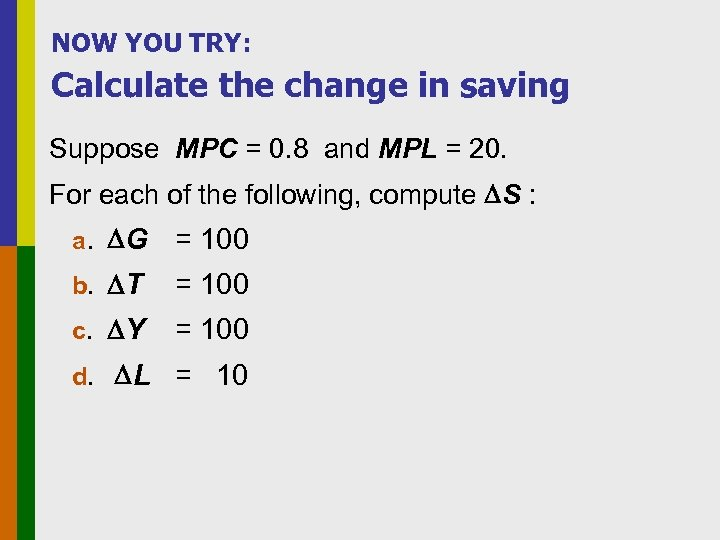NOW YOU TRY: Calculate the change in saving Suppose MPC = 0. 8 and MPL = 20. For each of the following, compute S : a. G = 100 b. T = 100 c. Y = 100 d. L = 10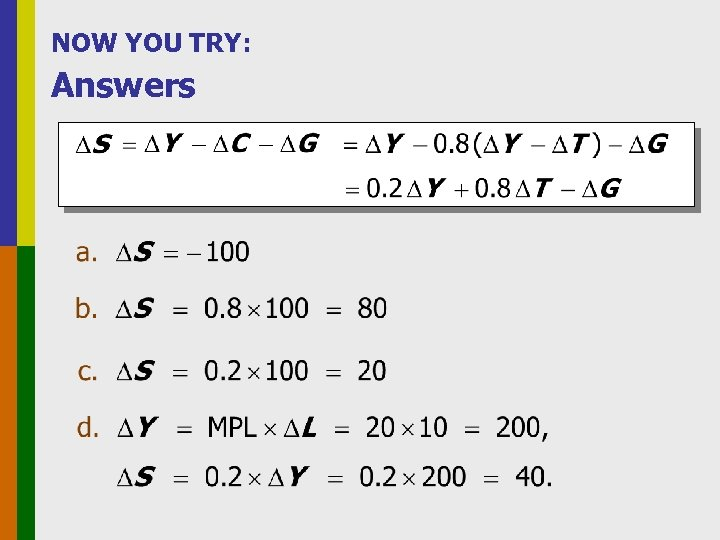NOW YOU TRY: Answers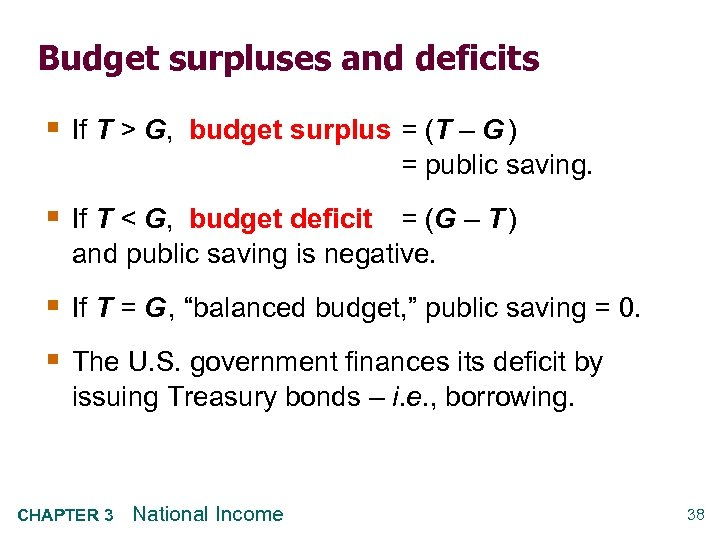Budget surpluses and deficits § If T > G, budget surplus = (T – G ) = public saving. § If T < G, budget deficit = (G – T ) and public saving is negative. § If T = G , “balanced budget, ” public saving = 0. § The U. S. government finances its deficit by issuing Treasury bonds – i. e. , borrowing. CHAPTER 3 National Income 38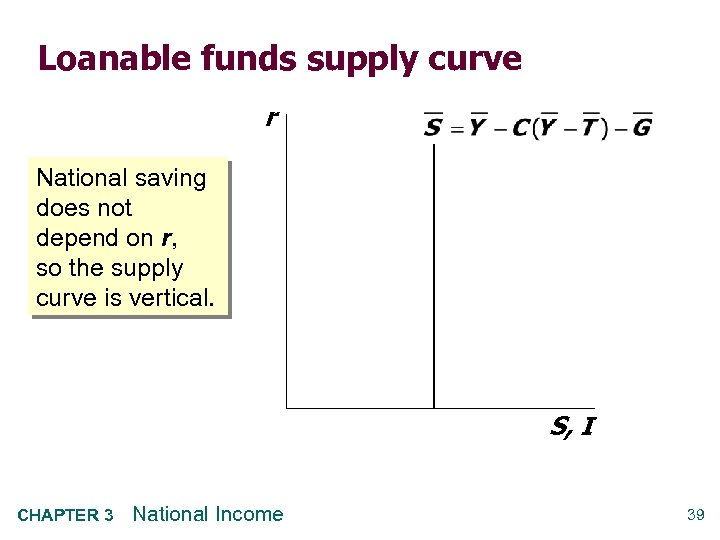Loanable funds supply curve r National saving does not depend on r, so the supply curve is vertical. S, I CHAPTER 3 National Income 39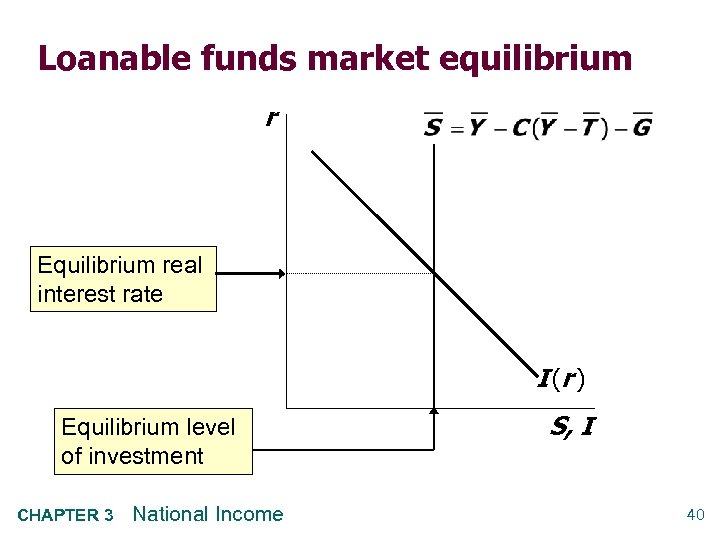Loanable funds market equilibrium r Equilibrium real interest rate I (r ) Equilibrium level of investment CHAPTER 3 National Income S, I 40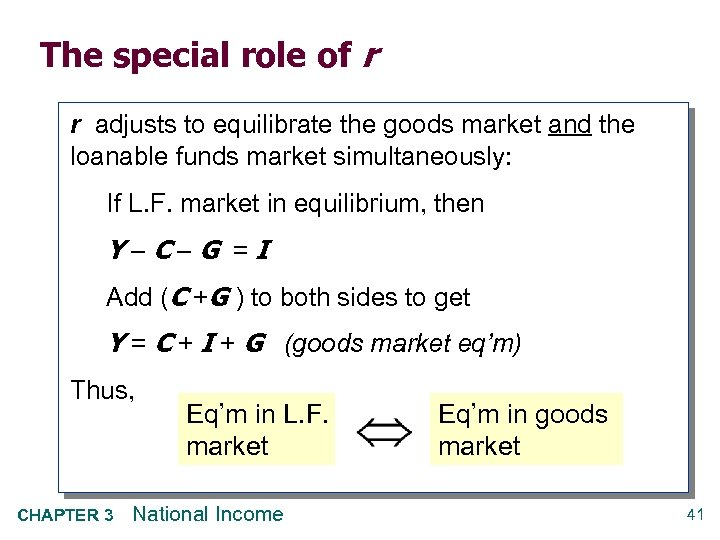The special role of r r adjusts to equilibrate the goods market and the loanable funds market simultaneously: If L. F. market in equilibrium, then Y–C–G =I Add (C +G ) to both sides to get Y = C + I + G (goods market eq’m) Thus, CHAPTER 3 Eq’m in L. F. market National Income Eq’m in goods market 41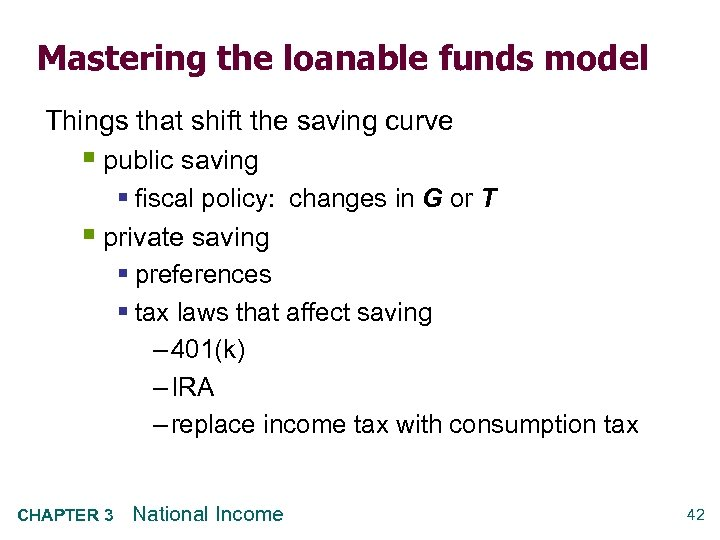Mastering the loanable funds model Things that shift the saving curve § public saving § fiscal policy: changes in G or T § private saving § preferences § tax laws that affect saving – 401(k) – IRA – replace income tax with consumption tax CHAPTER 3 National Income 42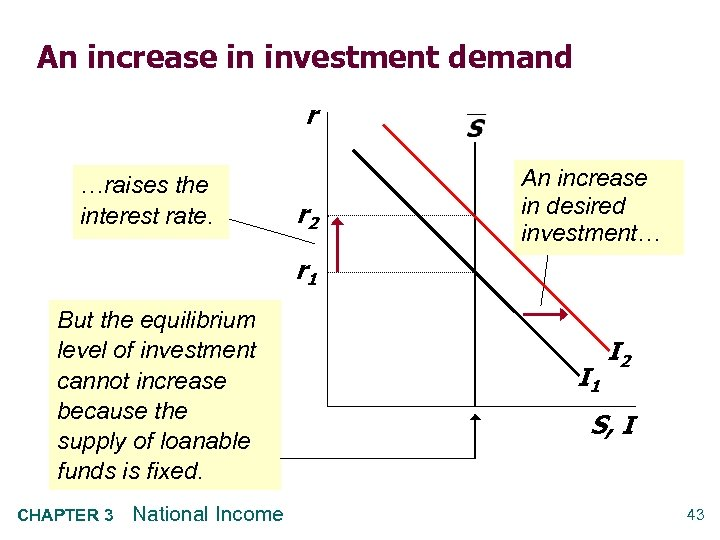An increase in investment demand r …raises the interest rate. r 2 An increase in desired investment… r 1 But the equilibrium level of investment cannot increase because the supply of loanable funds is fixed. CHAPTER 3 National Income I 1 I 2 S, I 43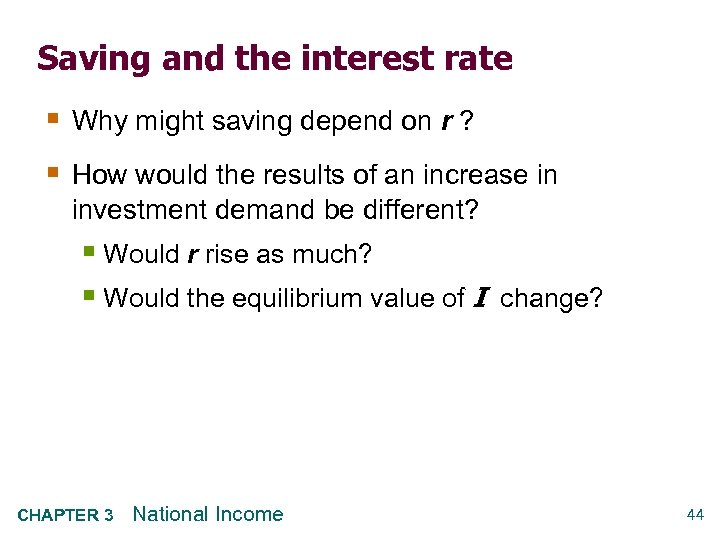Saving and the interest rate § Why might saving depend on r ? § How would the results of an increase in investment demand be different? § Would r rise as much? § Would the equilibrium value of I change? CHAPTER 3 National Income 44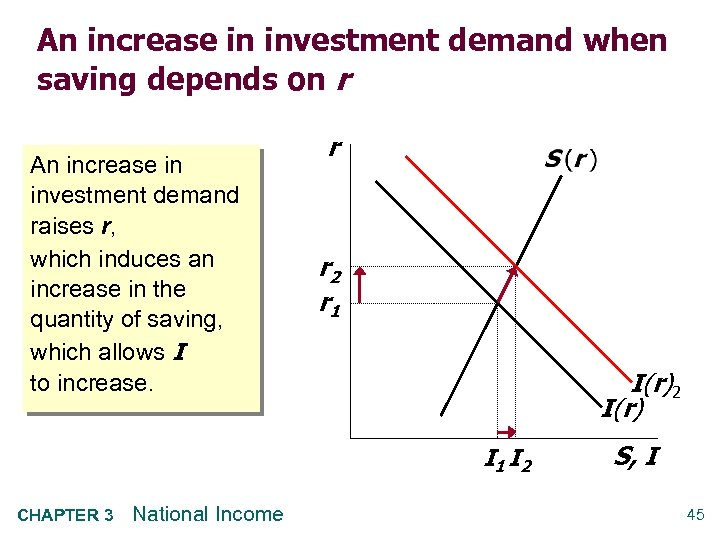An increase in investment demand when saving depends on r An increase in investment demand raises r, which induces an increase in the quantity of saving, which allows I to increase. r r 2 r 1 I(r)2 I(r) I 1 I 2 CHAPTER 3 National Income S, I 45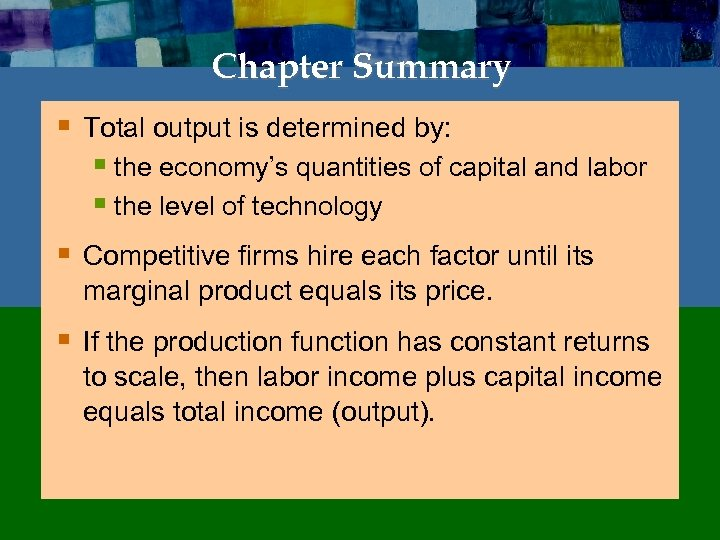Chapter Summary § Total output is determined by: § the economy’s quantities of capital and labor § the level of technology § Competitive firms hire each factor until its marginal product equals its price. § If the production function has constant returns to scale, then labor income plus capital income equals total income (output).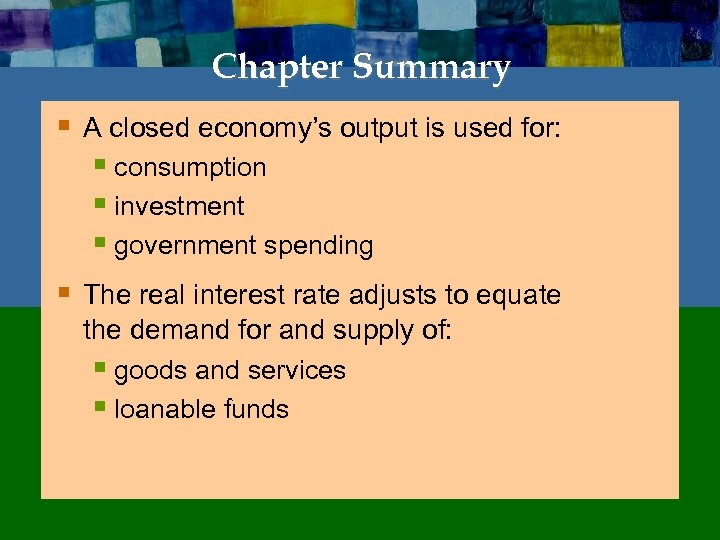Chapter Summary § A closed economy’s output is used for: § consumption § investment § government spending § The real interest rate adjusts to equate the demand for and supply of: § goods and services § loanable funds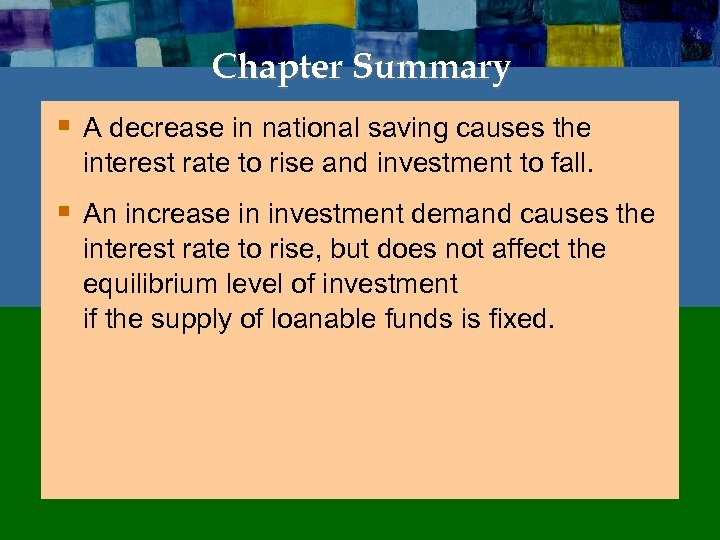Chapter Summary § A decrease in national saving causes the interest rate to rise and investment to fall. § An increase in investment demand causes the interest rate to rise, but does not affect the equilibrium level of investment if the supply of loanable funds is fixed.